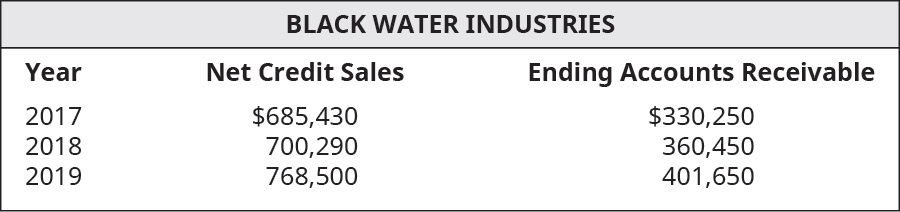# Using the following select financial statement information from Black Water Industries, compute the accounts receivable turnover ratios for 2018 and 2019 (round answers to two decimal places). What do the outcomes tell a potential investor about Black Water Industries?FindFindarrow_forward

### Principles of Accounting Volume 1

19th Edition
OpenStax
Publisher: OpenStax College
ISBN: 9781947172685

#### Solutions

Chapter
SectionFindFindarrow_forward

### Principles of Accounting Volume 1

19th Edition
OpenStax
Publisher: OpenStax College
ISBN: 9781947172685
Chapter 9, Problem 8EA
Textbook Problem
1 views

## Using the following select financial statement information from Black Water Industries, compute the accounts receivable turnover ratios for 2018 and 2019 (round answers to two decimal places). What do the outcomes tell a potential investor about Black Water Industries?To determine

Concept introduction:

Accounts receivable turnover ratio:

This is an efficiency ratio that indicates the conversion of accounts receivable into cash. This ratio is calculated by dividing the Net credit Sales by the Average accounts receivable. The formula to calculate this ratio is as follows:

Accounts receivable turnover ratio = Net credit SalesAverage accounts receivable

To indicate:

The Accounts receivable turnover ratio for each year and outcomes for a potential investor.

### Explanation of Solution

The Accounts receivable turnover ratio for each year is calculated as follows:

 Year 2018 Year 2019 Net Credit Sales (A) $700,290$ 768,500 Beginning Accounts Receivable (B) \$ 330,250

### Still sussing out bartleby?

Check out a sample textbook solution.

See a sample solution

#### The Solution to Your Study Problems

Bartleby provides explanations to thousands of textbook problems written by our experts, many with advanced degrees!

Get Started